vn.py官网

#### 配置数据库

vn.py 中默认使用 SQLite 数据库。 因此，如果需要使用 MongoDB 数据库，则需要修改 vn.py 的全局配置。具体流程如下：

1. `C:\Users\你的用户名\.vntrader` 目录下找到 `vt_setting.json` 文件

2. `vt_setting.json` 文件中的`database.driver,database.database,database.host,database.port` 进行修改。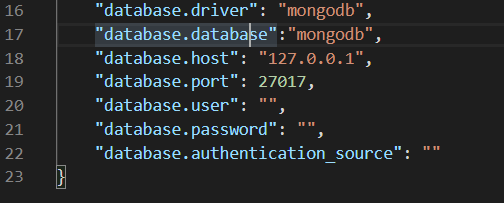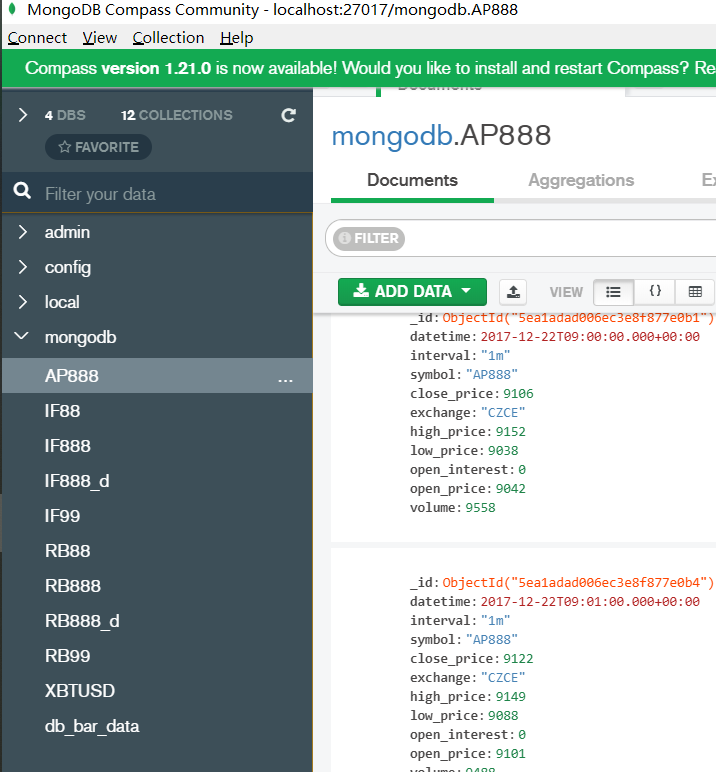#### 数据入库基本流程

1. 先确定要入库的数据是 `Tick` 还是 `Bar` (K线) 类型数据

2. 将需要入库的数据转化成 vn.py 定义的 `TickData``BarData` 数据类型

3. 使用 vn.py 提供的数据入库工具函数 `database_manager.save_tick_data``database_manager.save_bar_data` 将相应的 `TickData``BarData` 入库

#### 数据入库具体实现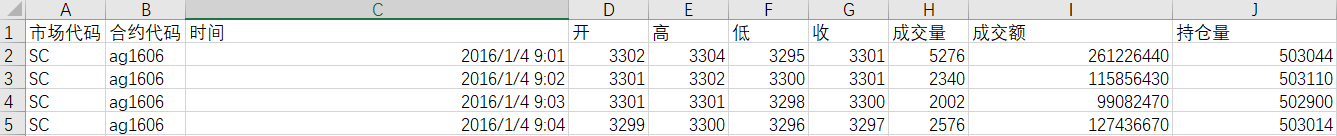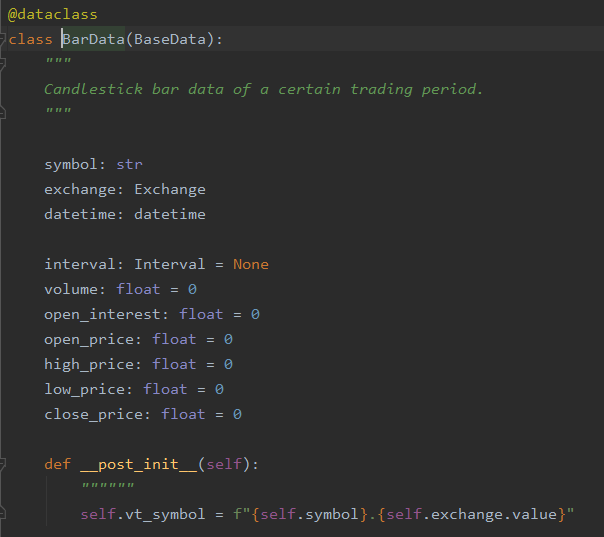`Exchange` 数据结构的代码：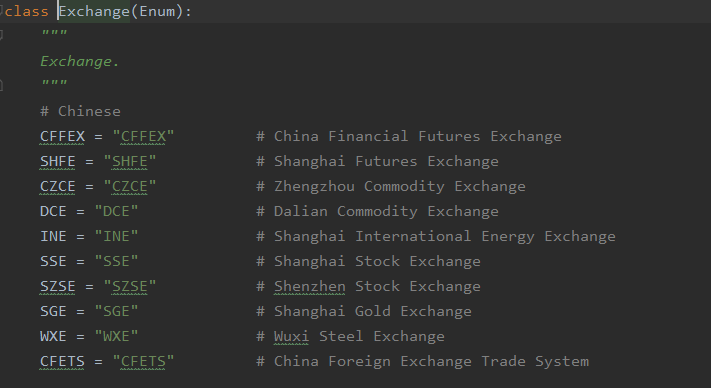`Interval` 数据结构的代码：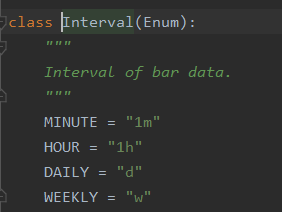1. csv文件中的 `合约代码 时间 开 高 低 收 成交量 持仓量``BarData`中的 `symbol datetime open_price high_price low_price close_price volume open_interest` 一一对应(从名称就就可以看出)。

2. csv文件中 `市场代码` 没办法和 `BarData` 中的 `exchange` 对应。因为csv文件中 `市场代码` 都是 `SC` ，而在上图`Exchange` 数据结构代码截图中找不到和 SC 对应的枚举常量的绑定值。从合约代码 ag1608(沪银1608) 可以推断出这里的 SC 指的就是上海期货交易所，对应的枚举常量是 `Exchang.SHFE`

3. csv文件中缺少了和 `BarData` 中的 `interval` 相对应的数据。上文我们已经发现了 csv文件中储存的是1分钟的`BarData`，对应的枚举常量是 `Interval.MINUTE`

1. 将csv文件中 `市场代码``SC` 替换成 `Exchang.SHFE`

2. 增加一列数据，且该列数据的所有值都是 `Interval.MINUTE`

```from vnpy.trader.constant import (Exchange, Interval)
import pandas as pd
# 读取需要入库的csv文件，该文件是用gbk编码

# 将csv文件中 `市场代码`的 SC 替换成 Exchange.SHFE SHFE
imported_data['市场代码'] = Exchange.SHFE

# 增加一列数据 `inteval`，且该列数据的所有值都是 Interval.MINUTE
imported_data['interval'] = Interval.MINUTE
```

```# 明确需要是float数据类型的列
float_columns = ['开', '高', '低', '收', '成交量', '持仓量']

for col in float_columns:
imported_data[col] = imported_data[col].astype('float')
```

```# 明确时间戳的格式
# %Y/%m/%d %H:%M:%S 代表着你的csv数据中的时间戳必须是 2020/05/01 08:32:30 格式
datetime_format = '%Y%m%d %H:%M:%S'

imported_data['时间'] = pd.to_datetime(imported_data['时间'],format=datetime_format)
```

```# 因为没有用到 成交额 这一列的数据，所以该列列名不变
imported_data.columns = ['exchange','symbol','datetime','open','high','low','close','volume','成交额','open_interest','interval']
```

```imported_data['symbol'] ='ag88'
```

```# 导入 database_manager 模块
# 封装函数
def move_df_to_mongodb(imported_data:pd.DataFrame,collection_name:str):
bars = []
start = None
count = 0

for row in imported_data.itertuples():

bar = BarData(

symbol=row.symbol,
exchange=row.exchange,
datetime=row.datetime,
interval=row.interval,
volume=row.volume,
open_price=row.open,
high_price=row.high,
low_price=row.low,
close_price=row.close,
open_interest=row.open_interest,
gateway_name="DB",

)

bars.append(bar)

# do some statistics
count += 1
if not start:
start = bar.datetime
end = bar.datetime

# insert into database
database_manager.save_bar_data(bars, collection_name)
print(f"Insert Bar: {count} from {start} - {end}")
```

```database_manager.save_bar_data(bars)
```

1. 创建一个sqlite数据库连接对象：

```from vnpy.trader.database.initialize import init_sql

settings={

"database": "database.db",
"host": "localhost",
"port": 3306,
"user": "root",
}
sqlite_manager = init_sql(driver=Driver.SQLITE, settings=settings)
```

2.使用sqlite数据库连接对象将数据入库

```# 替换函数 move_df_to_mongodb 的倒数第二行
sqlite_manager.save_bar_data(bars)
```

#### Debug

1. 找到`imported_data['时间'] = pd.to_datetime(imported_data['时间'],format=datetime_format)`代码所在行
2. 在该行代码下键入`imported_data['时间'] = imported_data['时间'].dt.strftime('%Y%m%d %H:%M:%S')`

#### 完整代码

```from vnpy.trader.constant import (Exchange, Interval)
import pandas as pd

# 封装函数
def move_df_to_mongodb(imported_data:pd.DataFrame,collection_name:str):
bars = []
start = None
count = 0

for row in imported_data.itertuples():

bar = BarData(

symbol=row.symbol,
exchange=row.exchange,
datetime=row.datetime,
interval=row.interval,
volume=row.volume,
open_price=row.open,
high_price=row.high,
low_price=row.low,
close_price=row.close,
open_interest=row.open_interest,
gateway_name="DB",

)

bars.append(bar)

# do some statistics
count += 1
if not start:
start = bar.datetime
end = bar.datetime

# insert into database
database_manager.save_bar_data(bars, collection_name)
print(f'Insert Bar: {count} from {start} - {end}')

if __name__ == "__main__":

# 读取需要入库的csv文件，该文件是用gbk编码
# 将csv文件中 `市场代码`的 SC 替换成 Exchange.SHFE SHFE
imported_data['市场代码'] = Exchange.SHFE
# 增加一列数据 `inteval`，且该列数据的所有值都是 Interval.MINUTE
imported_data['interval'] = Interval.MINUTE
# 明确需要是float数据类型的列
float_columns = ['开', '高', '低', '收', '成交量', '持仓量']
for col in float_columns:
imported_data[col] = imported_data[col].astype('float')
# 明确时间戳的格式
# %Y/%m/%d %H:%M:%S 代表着你的csv数据中的时间戳必须是 2020/05/01 08:32:30 格式
datetime_format = '%Y%m%d %H:%M:%S'
imported_data['时间'] = pd.to_datetime(imported_data['时间'],format=datetime_format)
# 因为没有用到 成交额 这一列的数据，所以该列列名不变
imported_data.columns = ['exchange','symbol','datetime','open','high','low','close','volume','成交额','open_interest','interval']
imported_data['symbol'] ='ag88'
move_df_to_mongodb(imported_data,'ag88')
```

1. 找到`imported_data['时间'] = pd.to_datetime(imported_data['时间'],format=datetime_format)`代码
2. 在上述代码下增加`imported_data['时间'] = imported_data['时间'].dt.strftime('%Y%m%d %H:%M:%S')`

imported_data['时间'] = imported_data['时间'].dt.strftime('%Y%m%d %H:%M:%S')

dt就是pands.series.dt.strftime的dt,官方文档在https://pandas.pydata.org/pandas-docs/stable/reference/api/pandas.Series.dt.strftime.html?highlight=dt%20strft#pandas.Series.dt.strftime

up

2020/09/09更新

```bar = BarData(

symbol=row.symbol,
exchange=row.exchange,
datetime=row.datetime,#报错的地方，需要增加时区信息
interval=row.interval,
volume=row.volume,
open_price=row.open,
high_price=row.high,
low_price=row.low,
close_price=row.close,
open_interest=row.open_interest,
gateway_name="DB",

)
```

`AttributeError: 'str' object has no attribute 'astimezone'`

```from datetime import datetime, timedelta, timezone

# 中国时区是+8，对应参数hours=8
# 日本时区是+9，hours=9
utc_8 = timezone(timedelta(hours=8))
datetime=row.datetime.replace(tzinfo=utc_8)
```

```sql_manager.get_oldest_bar_data()
```

callingpulse wrote:

2020/09/09更新

```bar = BarData(

symbol=row.symbol,
exchange=row.exchange,
datetime=row.datetime,#报错的地方，需要增加时区信息
interval=row.interval,
volume=row.volume,
open_price=row.open,
high_price=row.high,
low_price=row.low,
close_price=row.close,
open_interest=row.open_interest,
gateway_name="DB",

)
```

`AttributeError: 'str' object has no attribute 'astimezone'`

```from datetime import datetime, timedelta, timezone

# 中国时区是+8，对应参数hours=8
# 日本时区是+9，hours=9
utc_8 = timezone(timedelta(hours=8))
datetime=row.datetime.replace(tzinfo=utc_8)
```

```sql_manager.get_oldest_bar_data()
```

Traceback (most recent call last):
File "d:/study/论坛的入库程序.py", line 43, in <module>
move_df_to_mongodb(imported_data)
File "d:/study/论坛的入库程序.py", line 26, in move_df_to_mongodb
gateway_name="DB",
File "<string>", line 14, in init
File "D:\Python\Miniconda3\lib\site-packages\vnpy\trader\object.py", line 99, in post_init
self.vt_symbol = f"{self.symbol}.{self.exchange.value}"
AttributeError: 'str' object has no attribute 'value'

xiaohe wrote:

```
import pandas as pd

# 封装函数

def move_df_to_mongodb(imported_data: pd.DataFrame):
bars = []
start = None
count = 0

``````for row in imported_data.itertuples():

bar = BarData(
symbol=row.symbol,
exchange=row.exchange,
datetime=row.datetime,
interval=row.interval,
volume=row.volume,
open_price=row.open,
high_price=row.high,
low_price=row.low,
close_price=row.close,
open_interest=row.open_interest,
gateway_name="DB",
)
bars.append(bar)

# do some statistics
count += 1
if not start:
start = bar.datetime
end = bar.datetime

# insert into database
database_manager.save_bar_data(bars)
print(f'Insert Bar: {count} from {start} - {end}')

``````

if name == "main":
datatype = 1 # 1分钟数据用，2日线数据用

``````csvfilepath = 'e:\\test\\1test.csv'
# 读取需要入库的csv文件，该文件是用gbk编码
# 将csv文件中 `市场代码`的 SC 替换成 Exchange.SHFE SHFE
imported_data.loc[imported_data['exchange'].values == 'SSE', 'exchange'] = Exchange.SSE
imported_data.loc[imported_data['exchange'].values == 'SZSE', 'exchange'] = Exchange.SZSE
imported_data.loc[imported_data['exchange'].values == 'CFFEX', 'exchange'] = Exchange.CFFEX
# 增加一列数据 `inteval`，且该列数据的所有值都是 Interval.MINUTE
if datatype == 1:
imported_data['interval'] = Interval.MINUTE
elif datatype == 2:
imported_data['interval'] = Interval.DAILY
# 明确需要是float数据类型的列
float_columns = ['open', 'high', 'low', 'close', 'volume', 'open_interest']
for col in float_columns:
imported_data[col] = imported_data[col].astype('float')
# 明确时间戳的格式
# %Y/%m/%d %H:%M:%S 代表着你的csv数据中的时间戳必须是 2020/05/01 08:32:30 格式
datetime_format = '%Y/%m/%d %H:%M:%S'
imported_data['datetime'] = pd.to_datetime(imported_data['datetime'], format=datetime_format)
# 因为没有用到 成交额 这一列的数据，所以该列列名不变
imported_data.columns = ['exchange', 'symbol', 'name', 'datetime', 'open', 'high', 'low', 'close', 'volume',
'open_interest','interval']

move_df_to_mongodb(imported_data)
``````

```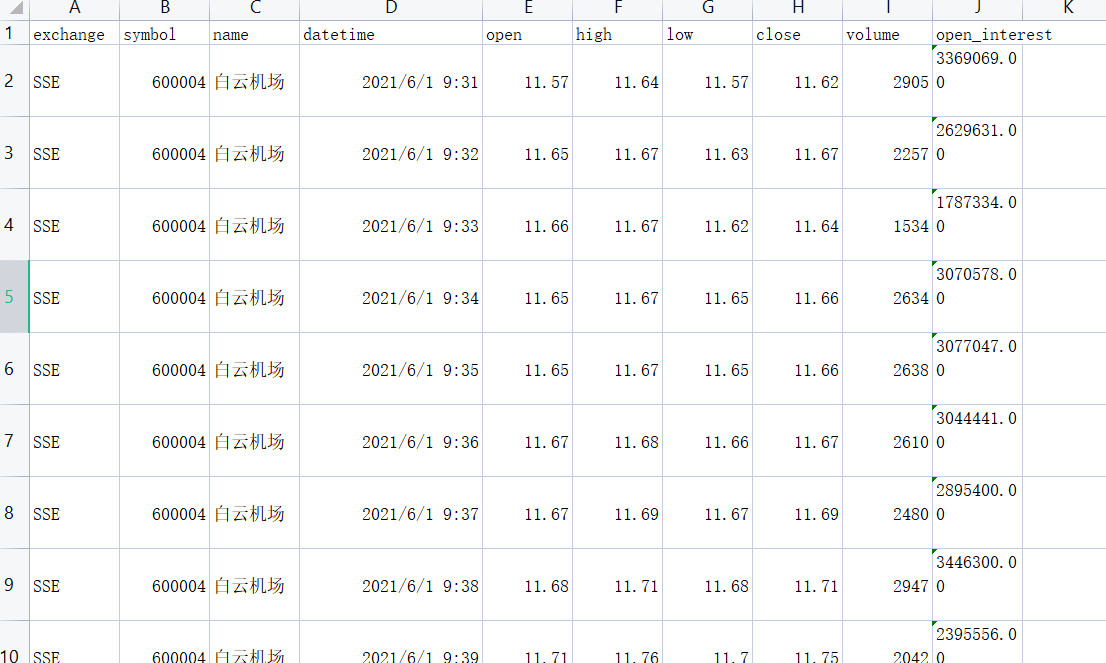VNPY的版本是2.3，现在出了
Traceback (most recent call last):
File "D:/study/论坛的入库程序.py", line 68, in <module>
move_df_to_mongodb(imported_data)
File "D:/study/论坛的入库程序.py", line 37, in move_df_to_mongodb
database_manager.save_bar_data(bars)
File "D:\Python\Miniconda3\lib\site-packages\vnpy\database\mongodb\mongodb_database.py", line 163, in save_bar_data
bar.datetime = convert_tz(bar.datetime)
File "D:\Python\Miniconda3\lib\site-packages\vnpy\trader\database.py", line 20, in convert_tz
dt = dt.astimezone(DB_TZ)
File "pandas/_libs/tslibs/timestamps.pyx", line 884, in pandas._libs.tslibs.timestamps.Timestamp.tz_convert
TypeError: Cannot convert tz-naive Timestamp, use tz_localize to localize

Name: datetime, Length: 3360, dtype: object

``````# %Y/%m/%d %H:%M:%S 代表着你的csv数据中的时间戳必须是 2020/05/01 08:32:30 格式
datetime_format = '%Y/%m/%d %H:%M:%S'
``````

"" move_df_to_mongodb(imported_data:pd.DataFrame,collection_name:str)""

ruzwdy wrote:

"" move_df_to_mongodb(imported_data:pd.DataFrame,collection_name:str)""